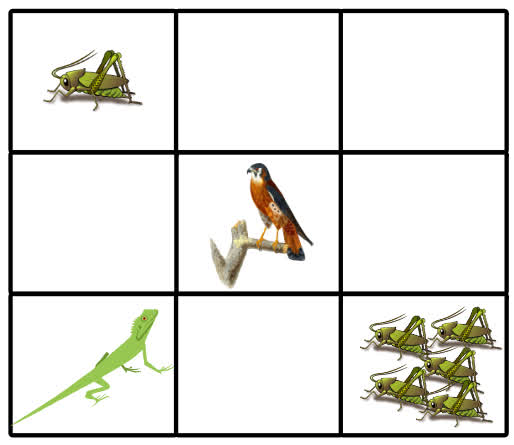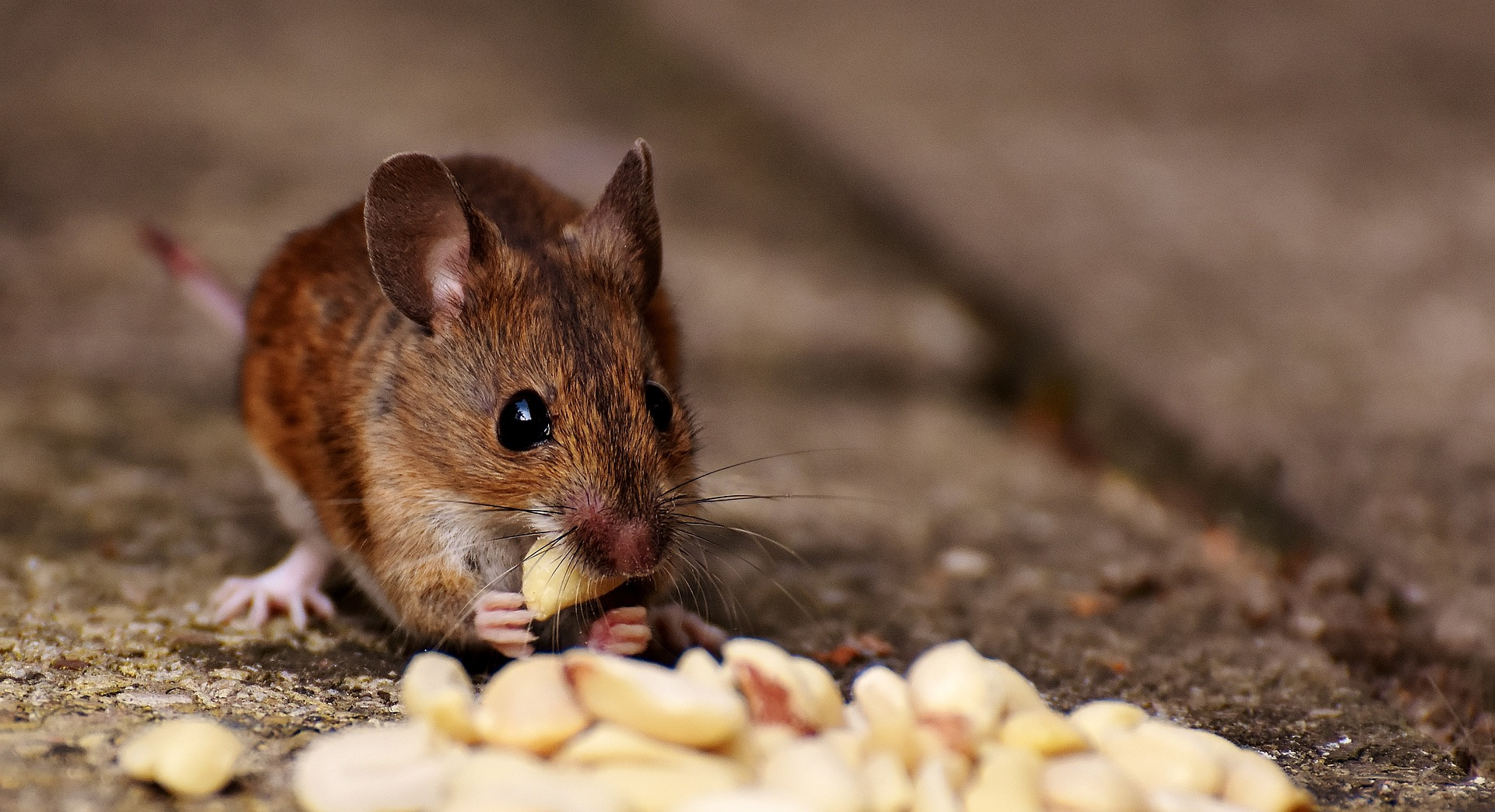# Reinforcement Learning - Goal Oriented Intelligence

Deep Learning Course 4 of 5 - Level: Advanced

## Exploration vs. Exploitation - Learning the Optimal Reinforcement Learning Policy

### video

expand_more chevron_left

### text

expand_more chevron_left

### Q-learning - Choosing actions with an epsilon greedy strategy

What's up, guys? Last time, we left our discussion of Q-learning with the question of how an agent chooses to either explore the environment or to exploit it in order to select its actions. To answer this question, we'll introduce a type of strategy called an epsilon greedy strategy, so let's get to it!Make sure you're up-to-speed on all that we've already introduced about Q-learning in the last post, and refresh your memory on where exactly we were in the example lizard game that we were using to illustrate Q-learning. We'll be picking up with that now.

### Review: The lizard game

Now, let's jump back into the lizard game.Remember, in each episode, the agent (the lizard in our case) starts out by choosing an action from the starting state based on the current Q-value estimates in the Q-table. The lizard chooses its action based on the highest Q-value for the given state.

Since we know that all of the Q-values are first initialized to zero, there's no way for the lizard to differentiate between them at the starting state of the first episode. So, the question remains, what action does it start with? Furthermore, for subsequent states, is it really as straight-forward as just selecting the action with the highest Q-value for the given state?

Additionally, we know that we need a balance of exploration and exploitation to choose our actions, but how exactly this is achieved is with an epsilon greedy strategy, so let's explore that now.

### Epsilon greedy strategy

To get this balance between exploitation and exploration, we use what is called an epsilon greedy strategy. With this strategy, we define an exploration rate $$\epsilon$$ that we initially set to $$1$$. This exploration rate is the probability that our agent will explore the environment rather than exploit it. With $$\epsilon=1$$, it is $$100\%$$ certain that the agent will start out by exploring the environment.

As the agent learns more about the environment, at the start of each new episode, $$\epsilon$$ will decay by some rate that we set so that the likelihood of exploration becomes less and less probable as the agent learns more and more about the environment. The agent will become “greedy” in terms of exploiting the environment once it has had the opportunity to explore and learn more about it.To determine whether the agent will choose exploration or exploitation at each time step, we generate a random number between $$0$$ and $$1$$. If this number is greater than epsilon, then the agent will choose its next action via exploitation, i.e. it will choose the action with the highest Q-value for its current state from the Q-table. Otherwise, its next action will be chosen via exploration, i.e. randomly choosing its action and exploring what happens in the environment.

if random_num > epsilon:
# choose action via exploitation
else:
# choose action via exploration


### Choosing an action

So, recall, we first started talking about the exploration-exploitation trade-off last time because we were discussing how the lizard should choose its very first action since all the actions have a Q-value of $$0$$.

Well now, we should know that the action will be chosen randomly via exploration since our exploration rate is set to $$1$$ initially. Meaning, with $$100\%$$ probability, the lizard will explore the environment during the first episode of the game, rather than exploit it.

Alright, so after the lizard takes an action, it observes the next state, the reward gained from its action, and updates the Q-value in the Q-table for the action it took from the previous state.

Let's suppose the lizard chooses to move right as its action from the starting state. We can see the reward we get in this new state is $$-1$$ since, recall, empty tiles have a reward of $$-1$$ point.

### Updating the Q-value

To update the Q-value for the action of moving right taken from the previous state, we use the Bellman equation that we highlighted previously:

\begin{eqnarray*} q_{\ast }\left( s,a\right) &=&E\left[ R_{t+1}+\gamma \max_{a^{\prime }}q_{\ast }\left( s^\prime,a^{\prime }\right)\right] \end{eqnarray*}

We want to make the Q-value for the given state-action pair as close as we can to the right hand side of the Bellman equation so that the Q-value will eventually converge to the optimal Q-value $$q_*$$.

This will happen over time by iteratively comparing the loss between the Q-value and the optimal Q-value for the given state-action pair and then updating the Q-value over and over again each time we encounter this same state-action pair to reduce the loss.

\begin{eqnarray*} q_{\ast }\left( s,a\right) - q(s,a)&=&loss \\E\left[ R_{t+1}+\gamma \max_{a^{\prime }}q_{\ast }\left( s^\prime,a^{\prime }\right)\right] - E\left[ \sum_{k=0}^{\infty }\gamma ^{k}R_{t+k+1}\right]&=&loss \end{eqnarray*}

To actually see how we update the Q-value, we first need to introduce the idea of a learning rate.

### The learning rate

The learning rate is a number between $$0$$ and $$1$$, which can be thought of as how quickly the agent abandons the previous Q-value in the Q-table for a given state-action pair for the new Q-value.

So, for example, suppose we have a Q-value in the Q-table for some arbitrary state-action pair that the agent has experienced in a previous time step. Well, if the agent experiences that same state-action pair at a later time step once it's learned more about the environment, the Q-value will need to be updated to reflect the change in expectations the agent now has for the future returns.

We don't want to just overwrite the old Q-value, but rather, we use the learning rate as a tool to determine how much information we keep about the previously computed Q-value for the given state-action pair versus the new Q-value calculated for the same state-action pair at a later time step. We'll denote the learning rate with the symbol $$\alpha$$, and we'll arbitrarily set $$\alpha = 0.7$$ for our lizard game example.

The higher the learning rate, the more quickly the agent will adopt the new Q-value. For example, if the learning rate is $$1$$, the estimate for the Q-value for a given state-action pair would be the straight up newly calculated Q-value and would not consider previous Q-values that had been calculated for the given state-action pair at previous time steps.

### Calculating the new Q-value

The formula for calculating the new Q-value for state-action pair $$(s,a)$$ at time $$t$$ is this:

\begin{equation*} q^{new}\left( s,a\right) =\left( 1-\alpha \right) ~\underset{\text{old value} }{\underbrace{q\left( s,a\right) }\rule[-0.05in]{0in}{0.2in} \rule[-0.05in]{0in}{0.2in}\rule[-0.1in]{0in}{0.3in}}+\alpha \overset{\text{ learned value}}{\overbrace{\left( R_{t+1}+\gamma \max_{a^{^{\prime }}}q\left( s^{\prime },a^{\prime }\right) \right) }} \end{equation*}So, our new Q-value is equal to a weighted sum of our old value and the learned value. The old value in our case is $$0$$ since this is the first time the agent is experiencing this particular state-action pair, and we multiply this old value by $$(1 - \alpha)$$.

Our learned value is the reward the agent receives from moving right from the starting state plus the discounted estimate of the optimal future Q-value for the next state-action pair $$(s^\prime,a^\prime)$$ at time $$t+1$$. This entire learned value is then multiplied by our learning rate.

All of the math for this calculation of our concrete example state-action pair of moving right from the starting state is shown below. Suppose the discount rate $$\gamma=0.99$$. We have

\begin{eqnarray*} q^{new}\left( s,a\right) &=&\left( 1-\alpha \right) ~\underset{\text{old value}}{\underbrace{q\left( s,a\right) }\rule[-0.05in]{0in}{0.2in} \rule[-0.05in]{0in}{0.2in}\rule[-0.1in]{0in}{0.3in}}+\alpha \overset{\text{ new value}}{\overbrace{\left( R_{t+1}+\gamma \max_{a^{^{\prime }}}q\left( s^{\prime },a^{\prime }\right) \right) }} \\ &=&\left( 1-0.7\right) \left( 0\right) +0.7\left( -1+0.99\left( \max_{a^{^{\prime }}}q\left( s^{\prime },a^{\prime }\right) \right) \right) \end{eqnarray*}

Let's pause for a moment and focus on the term $$\max_{a^{^{\prime }}}q\left( s^{\prime },a^{\prime }\right)$$. Since all the Q-values are currently initialized to $$0$$ in the Q-table, we have

\begin{eqnarray*} \max_{a^{^{\prime }}}q\left( s^{\prime },a^{\prime }\right) &=&\max \left( q\left( \text{empty6, left}\right),q\left( \text{empty6, right}\right),q\left( \text{empty6, up}\right),q\left( \text{empty6, down}\right) \right) \\ &=&\max \left( 0\rule[-0.05in]{0in}{0.2in},0,0,0\right) \\ &=&0 \end{eqnarray*}

Now, we can substitute the value $$0$$ in for $$\max_{a^{^{\prime }}}q\left( s^{\prime },a^{\prime }\right)$$ in our earlier equation to solve for $$q^{new}\left( s,a\right)$$.

\begin{eqnarray*} q^{new}\left( s,a\right) &=&\left( 1-\alpha \right) ~\underset{\text{old value}}{\underbrace{q\left( s,a\right) }\rule[-0.05in]{0in}{0.2in} \rule[-0.05in]{0in}{0.2in}\rule[-0.1in]{0in}{0.3in}}+\alpha \overset{\text{ new value}}{\overbrace{\left( R_{t+1}+\gamma \max_{a^{^{\prime }}}q\left( s^{\prime },a^{\prime }\right) \right) }} \\ &=&\left( 1-0.7\right) \left( 0\right) +0.7\left( -1+0.99\left( \max_{a^{^{\prime }}}q\left( s^{\prime },a^{\prime }\right) \right) \right) \\ &=&\left( 1-0.7\right) \left( 0\right) +0.7\left( -1+0.99\left( 0\right) \right) \\ &=&0+0.7\left( -1\right) \\ &=&-0.7 \end{eqnarray*}

Alright, so now we'll take this new Q-value we just calculated and store it in our Q-table for this particular state-action pair.

We've now done everything needed for a single time step. This same process will happen for each time step until termination in each episode.

Once the Q-function converges to the optimal Q-function, we will have our optimal policy.

### Max steps

Oh, and speaking of termination, we can also specify a max number of steps that our agent can take before the episode auto-terminates. With the way the game is set up right now, termination will only occur if the lizard reaches the state with five crickets or the state with the bird.

We could define some condition that states if the lizard hasn't reached termination by either one of these two states after $$100$$ steps, then terminate the game after the $$100^{th}$$ step.

### Wrapping up

Now, I know that was a lot, so I've summarized all of the steps for Q-learning we went through in the previous post and in this post! Again, go ahead and pause, check this out, let it simmer, and make sure you've got it.

1. Initialize all Q-values in the Q-table to $$0$$.
2. For each time-step in each episode:
• Choose an action ( considering the exploration-exploitation trade-off).
• Observe the reward and next state.
• Update the Q-value function ( using the formula we gave that will, overtime, make the Q-value function converge to the right hand side of the Bellman equation).In the next post, we're going to see how we can implement this Q-learning algorithm step-by-step in code using Python to play a simple game. We'll have lots more to talk about there.

Let me know in the comments if you're stoked to actually start getting your hands dirty to implement some of this stuff! And be sure to leave a thumbs up if you are. Thanks for contributing to collective intelligence, and I'll see ya in the next one!

### quiz

expand_more chevron_leftDEEPLIZARD Message notifications

### resources

expand_more chevron_left

expand_more chevron_leftDEEPLIZARD Message notifications